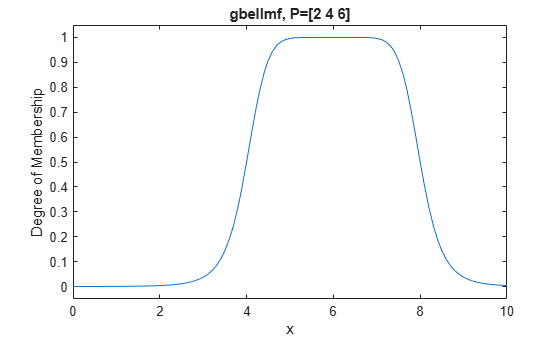# gbellmf

Generalized bell-shaped membership function

## Syntax

``y = gbellmf(x,params)``

## Description

This function computes fuzzy membership values using a generalized bell-shaped membership function. You can also compute this membership function using a `fismf` object. For more information, see fismf Object.

example

````y = gbellmf(x,params)` returns fuzzy membership values computed using the following generalized bell-shaped membership function:$f\left(x;a,b,c\right)=\frac{1}{1+{|\frac{x-c}{a}|}^{2b}}$To configure the membership function, specify parameters, a, b, and c using `params`.Membership values are computed for each input value in `x`.```

## Examples

collapse all

Specify input values across the universe of discourse.

`x = 0:0.1:10;`

Evaluate membership function for the input values.

`y = gbellmf(x,[2 4 6]);`

Plot the membership function.

```plot(x,y) title('gbellmf, P=[2 4 6]') xlabel('x') ylabel('Degree of Membership') ylim([-0.05 1.05])```## Input Arguments

collapse all

Input values for which to compute membership values, specified as a scalar or vector.

Membership function parameters, specified as the vector [a b c].

Here:

• a defines the width of the membership function, where a larger value creates a wider membership function.

• b defines the shape of the curve on either side of the central plateau, where a larger value creates a more steep transition.

• c defines the center of the membership function.

## Output Arguments

collapse all

Membership value returned as a scalar or a vector. The dimensions of `y` match the dimensions of `x`. Each element of `y` is the membership value computed for the corresponding element of `x`.

## Alternative Functionality

### `fismf` Object

You can create and evaluate a `fismf` object that implements the `gbellmf` membership function.

```mf = fismf("gbellmf",P); Y = evalmf(mf,X);```

Here, `X`, `P`, and `Y` correspond to the `x`, `params`, and `y` arguments of `gbellmf`, respectively.

## Version History

Introduced before R2006a

expand all